Practical Geometry
Serial order wise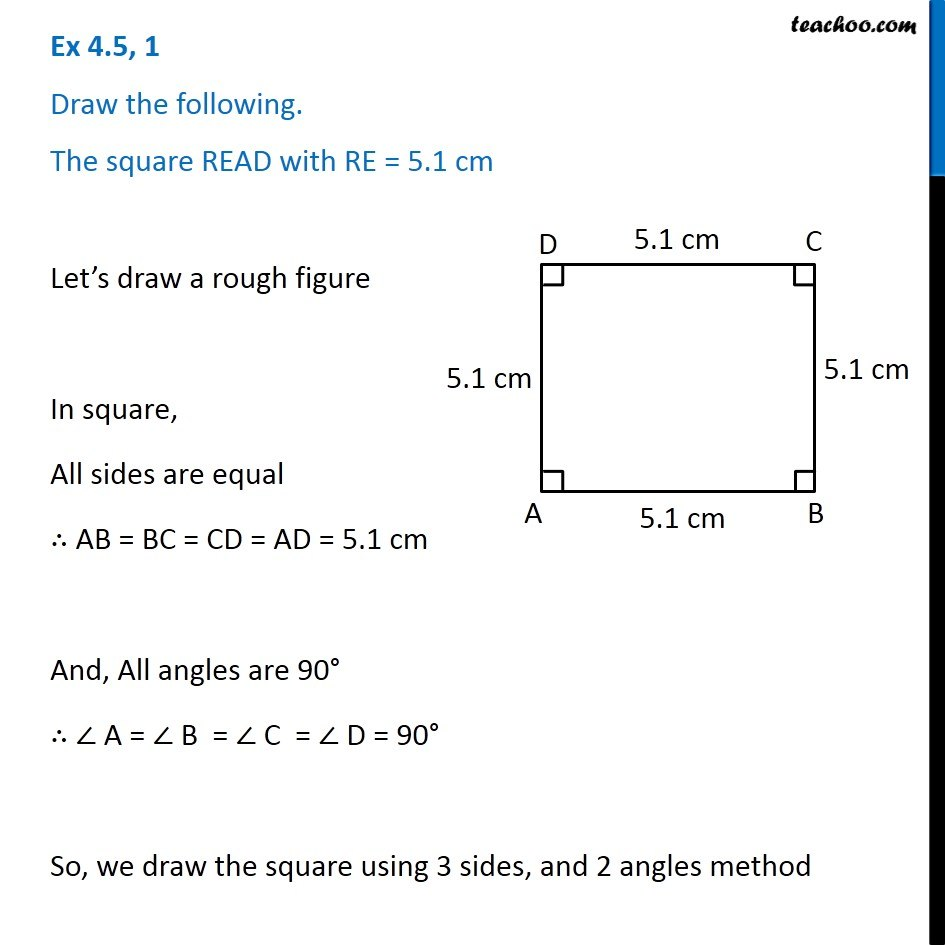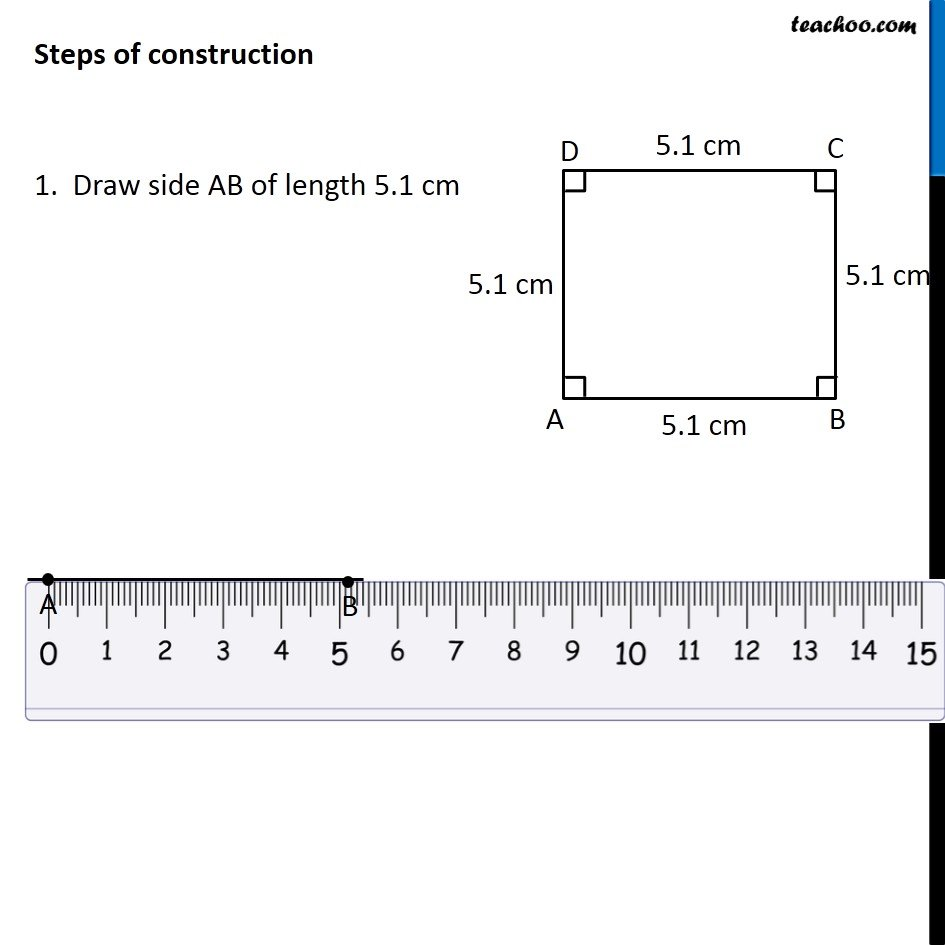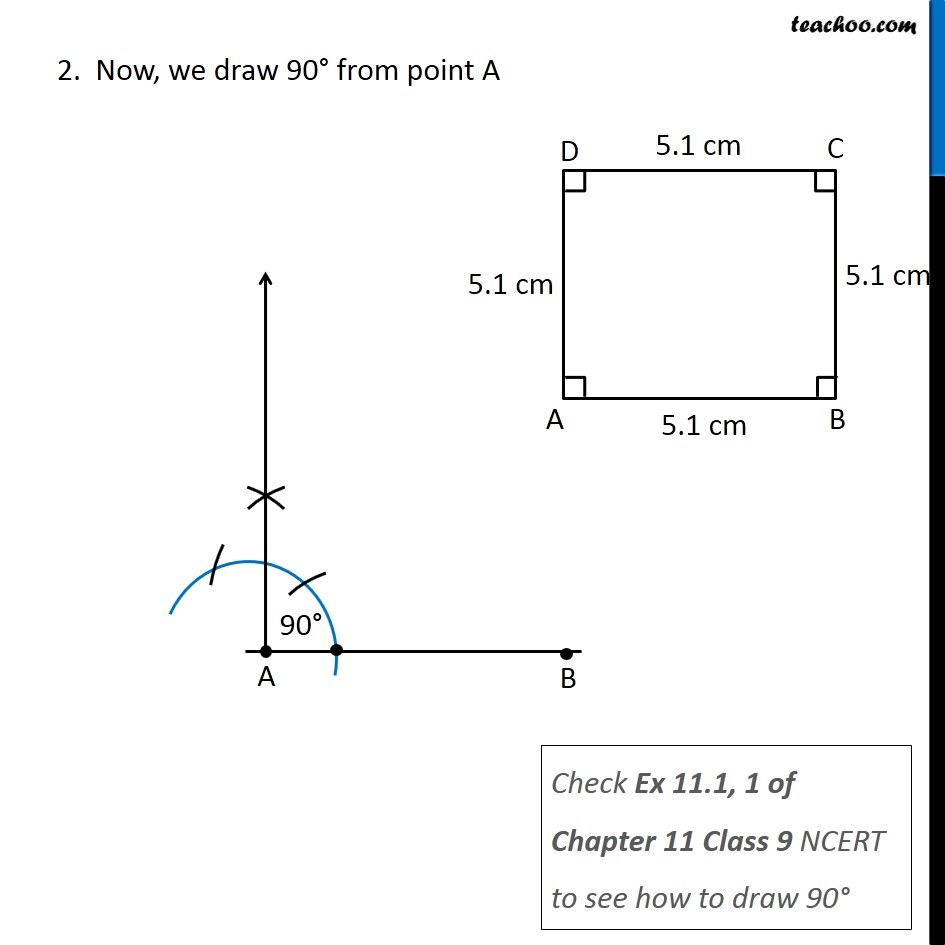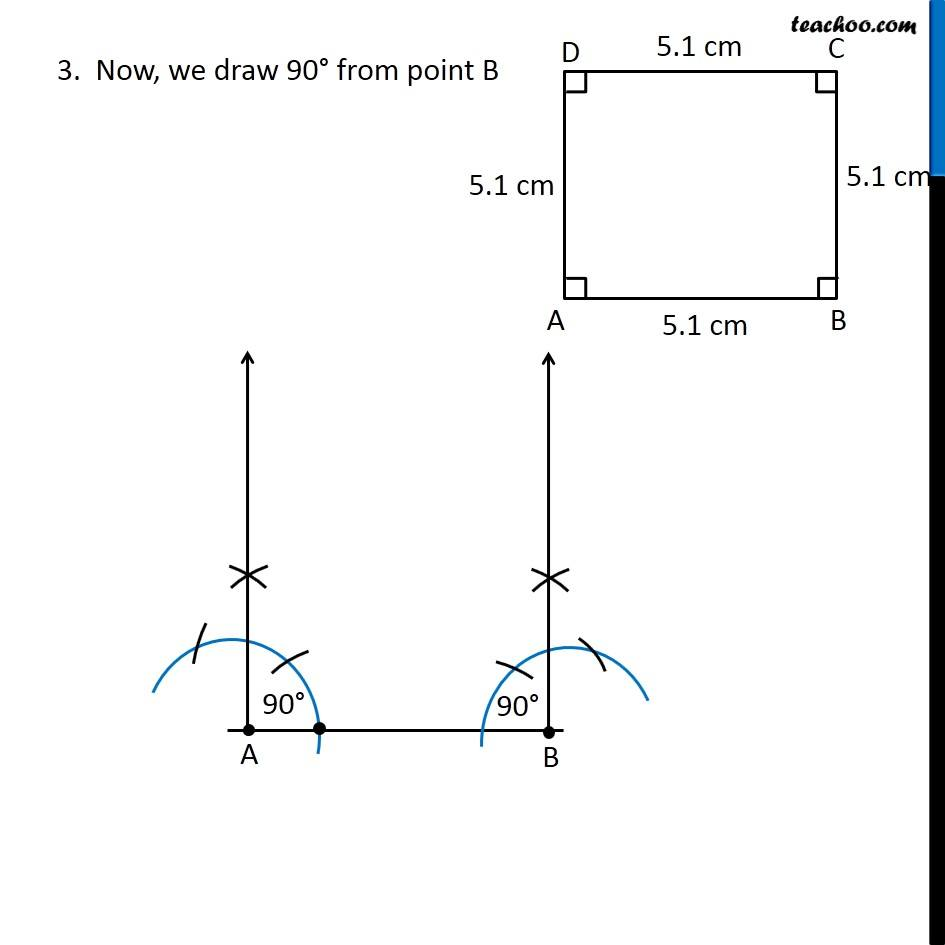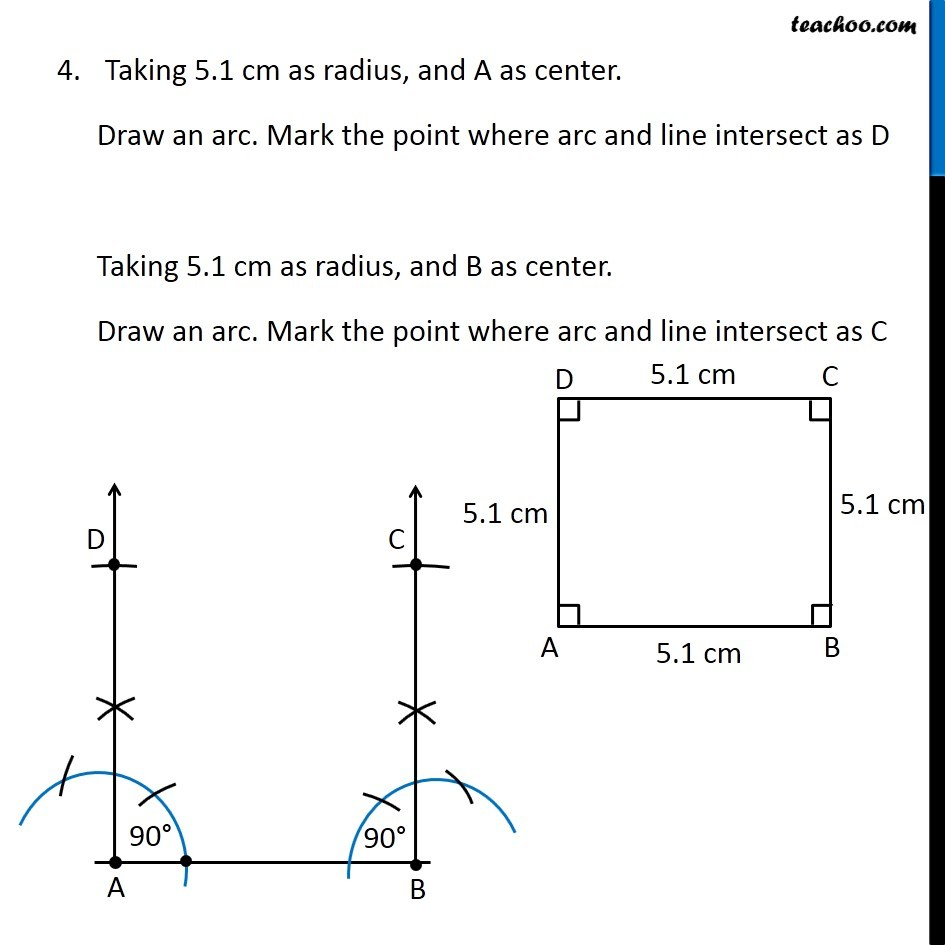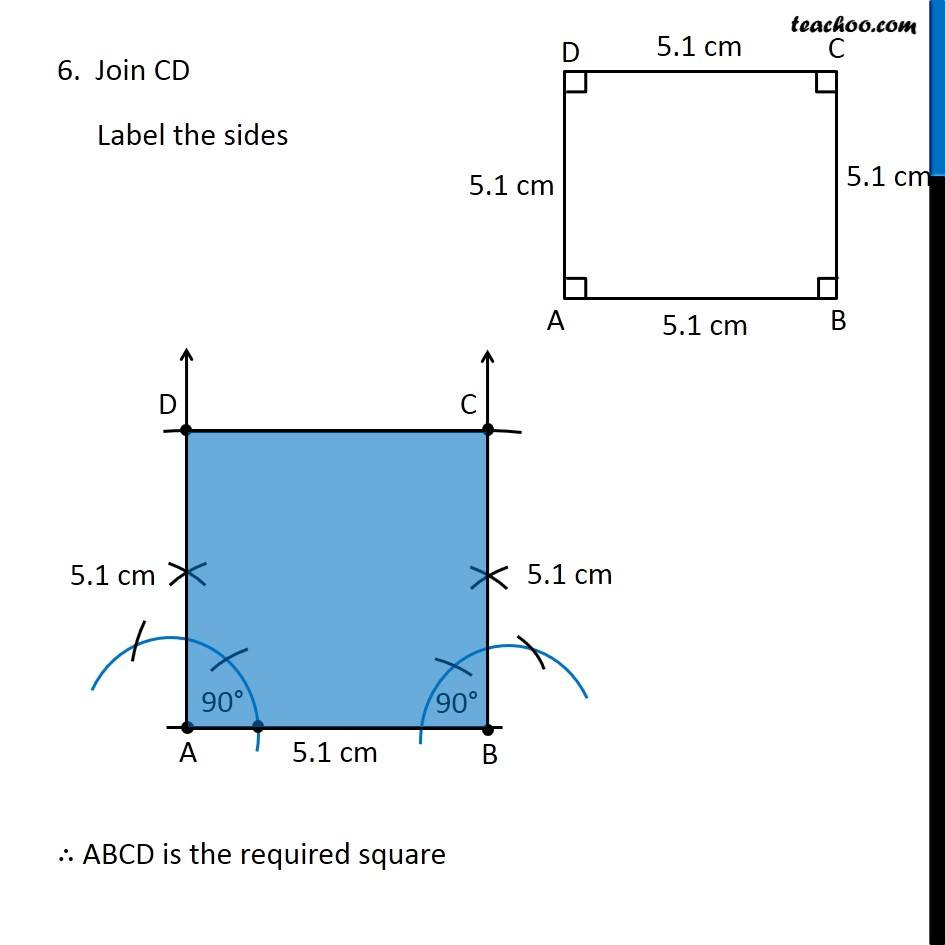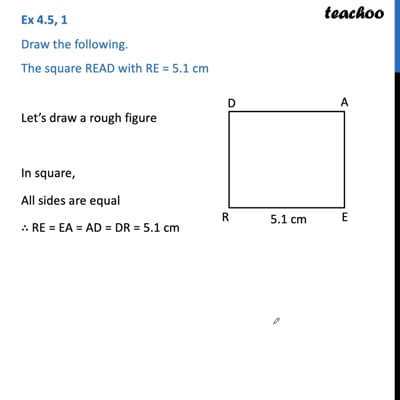This video is only available for Teachoo black users

Learn in your speed, with individual attention - Teachoo Maths 1-on-1 Class

### Transcript

Question 1 Draw the following. The square READ with RE = 5.1 cm Let’s draw a rough figure In square, All sides are equal ∴ AB = BC = CD = AD = 5.1 cm And, All angles are 90° ∴ ∠ A = ∠ B = ∠ C = ∠ D = 90° So, we draw the square using 3 sides, and 2 angles method Steps of construction 1. Draw side AB of length 5.1 cm 2. Now, we draw 90° from point A Check Ex 11.1, 1 of Chapter 11 Class 9 NCERT to see how to draw 90° 3. Now, we draw 90° from point B Taking 5.1 cm as radius, and A as center. Draw an arc. Mark the point where arc and line intersect as D Taking 5.1 cm as radius, and B as center. Draw an arc. Mark the point where arc and line intersect as C 6. Join CD Label the sides ∴ ABCD is the required square